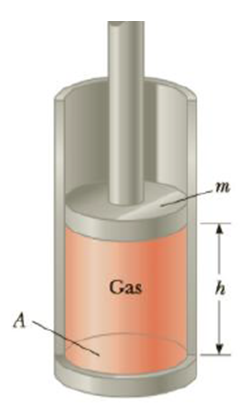Chapter 10, Problem 58AP

Chapter
Section
Textbook Problem

A vertical cylinder of cross-sectional area A is fitted with a tight-fitting, frictionless piston of mass m (Fig. P10.58). (a) If n moles of an ideal gas are in the cylinder at a temperature of T, use Newton’s second law for equilibrium to show that the height h at which the piston is in equilibrium under its own weight is given by h  =  n R T m g  +  P 0 A where P0 is the atmospheric pressure. (b) Is the pressure inside the cylinder less than, equal to, or greater than atmospheric pressure? (c) If the gas in the cylinder is warmed, how would the answer for h be affected?Figure P10.58

(a)

To determine
The height h.

Explanation

Given info: The number of moles of gas is n. The temperature is T. The cross sectional area is A.  Mass of the piston is m. The atmospheric pressure is P0 .

From Newton’s second law,

Fu=mg+Fd (I)

• g is the acceleration due to gravity.
• Fu is the upward force due to the absolute pressure of the gas inside.
• Fd is the downward force due to the atmospheric pressure.

Formula to calculate Fd is,

Fd=P0A (II)

Formula to calculate Fu is,

Fu=PA (III)

From Ideal gas equation, the absolute pressure of the gas is,

P=nRTV (IV)

• V is the volume

(b)

To determine
The pressure inside the cylinder.

(c)

To determine
The change in h when the gas is warmed.

Still sussing out bartleby?

Check out a sample textbook solution.

See a sample solution

The Solution to Your Study Problems

Bartleby provides explanations to thousands of textbook problems written by our experts, many with advanced degrees!

Get Started

Find more solutions based on key concepts